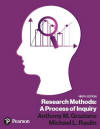﻿ PythagorousGraziano & Raulin
Research Methods (9th edition)

Pythagoras (ca 500 B.C.) was a Greek philosopher and mathematician. He had been instructed in the Ionian School (see Thales) and was the founder of the Pythagorean school of religion and philosophy. This was a mystical religious order that believed that the precision of, and relationships among, numbers showed the bases on which the universe was organized and on which it functions.

The Pythagoreans believed that celestial bodies, such as the sun, moon, and planets, are perfectly circular, and they move in circular paths in uniform ways, reflecting the perfection of the Gods. Such harmony in the universe, they believed, was closely related to numbers.

By elevating the importance of numbers and the value of studying them, the Pythagoreans actually elevated a religious mysticism to a mathematical science. In the process, they created the study of geometry. One of their mathematical discoveries was the Pythagorean theorem--that the square of the hypotenuse of a right triangle is equal to the sum of the squares of the other two sides. (It should be noted that some historians credit this discovery to the Egyptians, long before Pythagoras.)

The Pythagoreans took the Ionian school of philosophy to a far more abstract level and influenced the subsequent development of science and mathematics to the present day.

 Previous Page Name Index Help Period Index Next Page